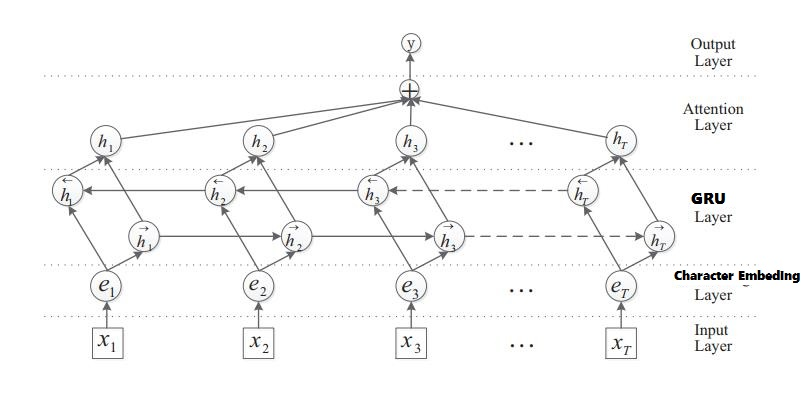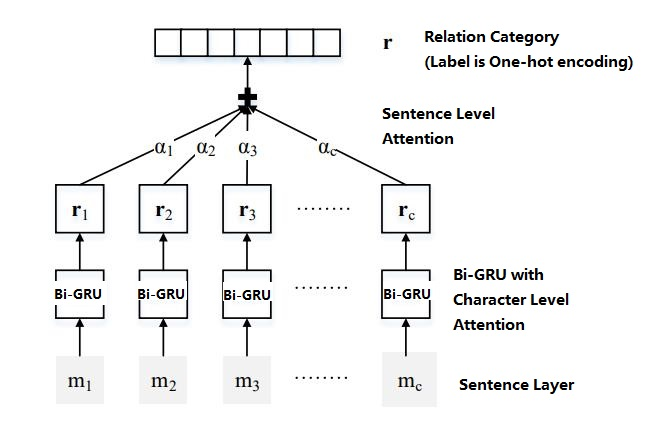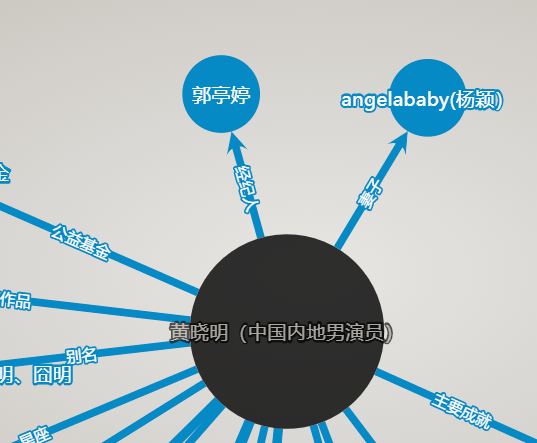## 双向GRU加Dual Attention模型## 语料获取## 模型训练

### 系统要求：

• Python (>=3.5) 一定要用Python 3。因为编码问题，Python 2对中文太不友好。

• TensorFlow (>=r1.0) Tensorflow几个月一个大变样，对<1.0暂不支持。不过清华Thunlp的原始代码是旧版的Tensorflow，对旧版TF仍然执迷不悟念念不忘的朋友们可以去参考。

• scikit-learn (>=0.18)

### 训练:

1.所有数据准备在 origin_data/ 中, 包括了关系种类 (relation2id.txt), 训练数据 (train.txt), 测试数据 (test.txt) and 中文字向量 (vec.txt). 中文字向量是在中文wikipedia上训练的。训练数据和测试数据是将原始数据随机排序，然后按大概80%做训练，20%做测试的方法分开。更好的方法是再加入验证数据，但是训练一个epoch已经好长时间，就偷个懒不搞k-fold什么的了。。。

``````现有的数据包含以下12种关系种类：
unknown, 父母, 夫妻, 师生, 兄弟姐妹, 合作, 情侣, 祖孙, 好友, 亲戚, 同门, 上下级
``````

2.将所有数据通过字向量整理成numpy的形式，存储在 data/

``````python initial.py
``````

3.进行训练，模型会存储在 model/。并没有怎么仔细调节超参数，模型结构除了字向量的输入以外，也是完全照搬英文版的模型。感兴趣的朋友们以这个结果为baseline可以尝试调出更好的模型参数。

``````python train_GRU.py
``````

### 预测：

``````python test_GRU.py
``````

## 一些结果

``````INFO:tensorflow:Restoring parameters from ./model/ATT_GRU_model-9000

No.1: 夫妻, Probability is 0.996217
No.2: 父母, Probability is 0.00193673
No.3: 兄弟姐妹, Probability is 0.00128172

No.1: 好友, Probability is 0.526823
No.2: 兄弟姐妹, Probability is 0.177491
No.3: 夫妻, Probability is 0.132977

No.1: 上下级, Probability is 0.965674
No.2: 亲戚, Probability is 0.0185355
No.3: 父母, Probability is 0.00953698

No.1: 祖孙, Probability is 0.785542
No.2: 好友, Probability is 0.0829895
No.3: 同门, Probability is 0.0728216

No.1: 师生, Probability is 0.735982
No.2: 同门, Probability is 0.159495
No.3: 兄弟姐妹, Probability is 0.0440367

No.1: 师生, Probability is 0.994964
No.2: 父母, Probability is 0.00460191
No.3: 夫妻, Probability is 0.000108601

No.1: 兄弟姐妹, Probability is 0.852632
No.2: 亲戚, Probability is 0.0477967
No.3: 好友, Probability is 0.0433101

No.1: 亲戚, Probability is 0.766272
No.2: 父母, Probability is 0.162108
No.3: 兄弟姐妹, Probability is 0.0623203

No.1: 合作, Probability is 0.907599
No.2: unknown, Probability is 0.082604
No.3: 上下级, Probability is 0.00730342

No.1: 合作, Probability is 0.819008
No.2: 上下级, Probability is 0.116768
No.3: 师生, Probability is 0.0448312
``````